IMO Shortlist 2015 problem G7

Kvaliteta:
Avg: 0,0
Težina:
Avg: 9,0

Let$ABCD$ be a convex quadrilateral, and let$P$,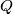$Q$,$R$, and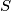$S$ be points on the sides$AB$,$BC$,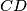$CD$, and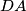$DA$, respectively. Let the line segment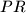$PR$ and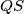$QS$ meet at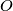$O$. Suppose that each of the quadrilaterals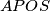$APOS$,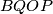$BQOP$,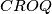$CROQ$, and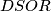$DSOR$ has an incircle. Prove that the lines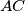$AC$,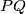$PQ$, and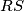$RS$ are either concurrent or parallel to each other.

(Bulgaria)

Izvor: https://www.imo-official.org/problems/IMO2015SL.pdf